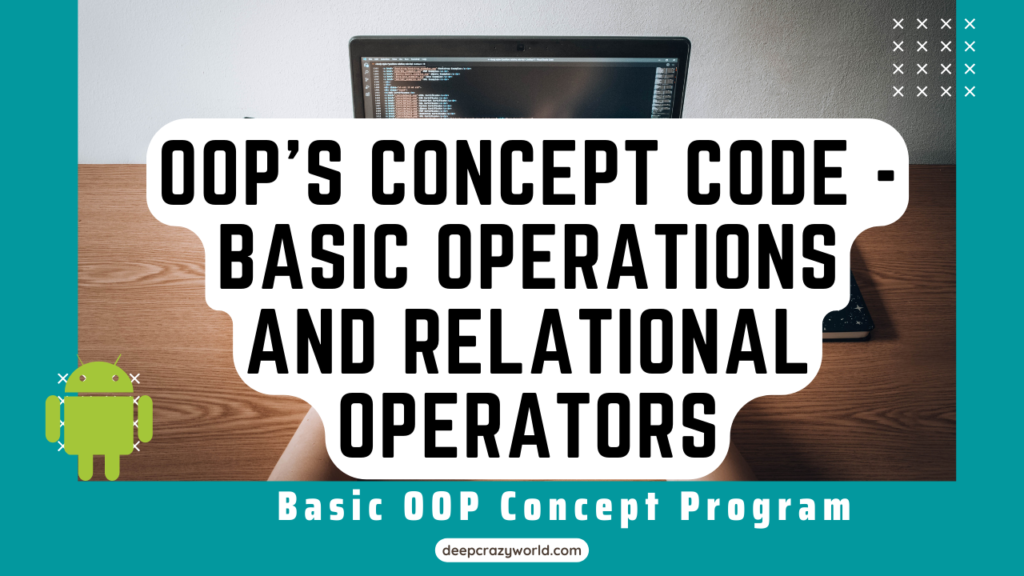# OOP’s Concept Code – Basic operations and Relational Operators

OOP’s Concept Code – Basic operations and Relational Operators 2022 – Object means a real-world entity such as a pen, chair, table, computer, watch, etc. Object-Oriented Programming is a methodology or paradigm to design a program using classes and objects. It simplifies software development and maintenance by providing some concepts:

• Object
• Class
• Inheritance
• Polymorphism
• Abstraction
• Encapsulation

Apart from these concepts, there are some other terms which are used in Object-Oriented design:

• Coupling
• Cohesion
• Association
• Aggregation
• Composition

## Basic operations

``````    Addition = +
Subtraction "-"
Multiplication "*"
Division "/"
Remainder = "what remains.." 4 % 2 = 0 because 2*2 = 4 and there's not remainder
4%3 = 1``````

## Relational Operators

``````        == comparison, not assignment
!= NOT equal
> greater than
< less than
>= Greater than or equal
<= Less than or equal``````

## Logical Operators

``````        AND ( && ) == both "sides" need to be TRUE in order for the whole expression to return true
OR ( || )
NOT ( ! )``````

## PROGRAM

``````package com.gohooljava.com;

public class Main {

public static void main(String[] args) {

/*
for loop
while loop
*/

int i = 0;

while (i < 5) {

System.out.println(" Counting.. " + i);
i++;

}

// Make sure to uncomment the code in order to be able to run the program successfully :)

//        for (int i = 0; i < 20; i++) {
//
//           //remainder ( %)
//            if ( i % 4 == 0) {
//
//                System.out.println( i + " is a multiple of 4 ");
//
//            }
//
//
//        }

//Basic operations
//Subtraction "-"
//Multiplication "*"
//Division "/"
//Remainder = "what remains.." 4 % 2 = 0 because 2*2 = 4 and there's not remainder
// 4%3 = 1

int firstNum1 = 34;
int secondNum2 = 3;
System.out.println("The sum is +" (firstNum1 + secondNum2)); // Always add parenthesis in order for this to work

// the "quotes are placed wrong as it gives the compilation error

//Subtraction
System.out.println("The result is +" (firstNum1 - secondNum2)); // Always add parenthesis in order for this to work

//Multiplication
System.out.println("The result is +" (firstNum1 * secondNum2));

//Division
System.out.println("The result is +" (firstNum1 / secondNum2));

// ==== Relational Operators ===
/*
- == comparison, not assignment
- != NOT equal
- > greater than
- < less than
- >= Greater than or equal
- <= Less than or equal
==== Logical Operators =====
- AND ( && ) == both "sides" need to be TRUE in order for the whole expression to return true
- OR ( || )
- NOT ( ! )
*/
//        double firstNum = 10;
//        double secondNum = 3;
//        double result = 0;
//
//        boolean isOld = true;
//        boolean isYoung = false;
//
//
//        if ( (isOld && !isYoung) && (firstNum < secondNum)  ) {
//
//            System.out.println("Horray!!");
//
//        }else {
//
//            System.out.println("Not right!");
//
//        }

//        result = firstNum % secondNum;
//
//        // If statements
//        if (firstNum <= secondNum) {
//
//
//            System.out.println("The remainder is " + result );
//
//        }else {
//
//            System.out.println("Numbers aren't equal");
//
//        }

}
}``````